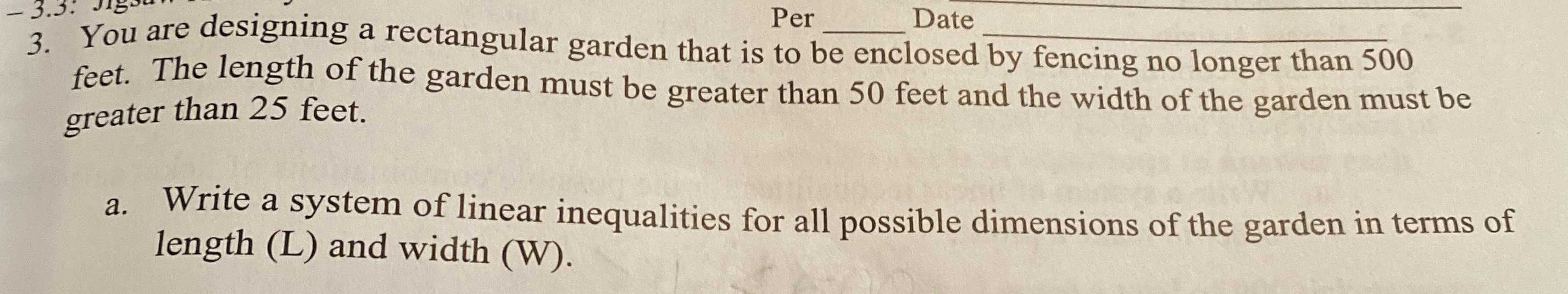### ¿Todavía tienes preguntas de matemáticas?

Pregunte a nuestros tutores expertos
Algebra
Pregunta3. You are designing a rectangular garden that is to be enclosed by fencing no longer than $$500$$ feet. The length of the garden must be greater than $$50$$ feet and the width of the garden must be greater than $$25$$ feet.

a. Write a system of linear inequalities for all possible dimensions of the garden in terms of length (L) and width (W).

2L+2W$$\le$$500
L$$\ge$$50
W$$\le$$25